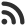Feed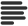Articles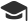Tutorials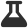CodingLab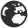CommunityLeaderboardFounder & Programmer at HackersFriend Updated July 9, 2019, 1:03 p.m. ⋅ 186 views

# Find Sum of all Submatrices of a Given Matrix

Problem

You are given a N * N 2D Matrix.

Your task is to find sum of all possible submatrices. Which can be produced from this matrix.

Have a look at input and output for better understanding.

Input

``arr[] = {{1, 1}, {1, 1}};``

Output

``16``

Explanatation

Sum of sub matrices with size 1 = 1*4 = 4

Sum of sub matrices with size 2 = 2*4 = 8

Similarly, Sum of sub matrices with size 3 = 3 * 0 (since, no 3 * 3 matrix is possible)  = 0

Sum of sub matrices with size 4 = 4*1 =4

Hence, total sum = 4 + 8 + 0 + 4 = 16.

Have a look at another example too.

Input

``arr[] = {{1, 2, 3},{4, 5, 6}, {7, 8, 9}}``

Output

``500``

Solution

Brute force - We can solve this question just by traversing the whole matrix N times and generating and calculating their sums.

But problem, is this is very in efficient way to solve this problem. Once, the size of matrix gets bigger, it will be very time consuming and start producing TLE in competitve programming. Time complexity of this soltuion will be O(n6).

Tricky Solution

Key here is to understand the relation. Let's beak it down.

We'll first find out, how many times an element of matirix can occur in submatrices.

Let's call this element Arr(x,y). Where x and y is position of element in 0 based indexing.

So total occurance of Arr(x,y) will be (X + 1) * (Y + 1) * (N – X) * (N – Y)

Let's call this total number of occurance be S(x,y).

So, total sum of produced by this element will be Arr(x,y) * S(x,y).

Now, we can figure out total sum of submatrices by adding total sum of all the elements of matrix.

Let's code it.

``````#include <iostream>
using namespace std;
int matrixSum(int arr[])
{

int sum = 0; // this will store total sum

// Find out number of submatrices, each element belongs to
for (int i = 0; i < n; i++)
for (int j = 0; j < n; j++) {

// Number of ways to choose
// from top-left elements
int top_left = (i + 1) * (j + 1);

// Number of ways to choose
// from bottom-right elements
int bottom_right = (n - i) * (n - j);
sum += (top_left * bottom_right * arr[i][j]);
}

return sum;
}

// Main Function
int main()
{
int arr = { { 1, 1, 1 },
{ 1, 1, 1 },
{ 1, 1, 1 } };

cout << matrixSum(arr);

return 0;
}
``````

This will produce the follwing output.

``100``

 arrow_upward UpvoteComment arrow_downward downvote

Events

Nov. 28, 2018, 5:30 p.m.

Python from zero to hero

place Delhi

Aug. 13, 2018, 5:30 p.m.

Python from zero to hero

place Bangalore ( HackersFriend office BTM Layout)